# Fourier transform, discrete

(diff) ← Older revision | Latest revision (diff) | Newer revision → (diff)
If a function is given on the set of pointsby its values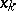,,, whereis the period of the function, then the discrete Fourier transform of the vector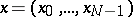is the vector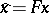, whereis the matrix with entries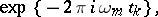is the imaginary unit,,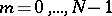,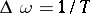. The components of the vectorare analogues of the Fourier coefficients in the usual trigonometric expansions. The discrete Fourier transform is used to calculate approximately these coefficients, spectra, auto- and mutual-correlation functions, etc. A direct computation of the discrete Fourier transform requires aboutarithmetic operations and a great expenditure of machine time. The method of the fast Fourier transform (see ) enables one to reduce the number of operations considerably. When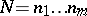, this method carries out the discrete Fourier transform approximately inoperations, increasing the accuracy of the calculation. When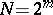there are algorithms which are particularly convenient in practice. There is a considerable number of programs realizing or using the fast Fourier transform to solve applied problems. The method of the fast Fourier transform includes widely known efficient methods for computing the discrete Fourier transform, for example, the Runge method (see  and Runge–Kutta method).